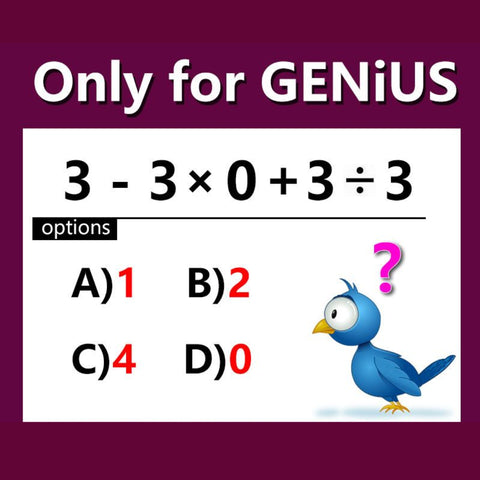Free Shipping On All Orders

## Viral Math Problems #57• The answer is 2. I know this because of PEMDAS. P is for parentheses and there are no parentheses. So then M for multiplication. 3 times 0 is 0. The D for division. 3 divided by 3 is 1. 1 plus 0 is 1. Then there is no addition but there is S for subtraction. So 3 minus 1 is 2. why do we do minus 1? We do it because it says 3 minus 3 times 0 plus 3 divided by 3 so whatever the answer for that is you minus it by 3.

Alessandra on

• It’s got to be 2. My father taught me PEMDAS. There are no parentheses. First comes multiplication and division. 3 × 0 = 0. 3 ÷ 3 = 1. 0 + 1 = 1. 3 – 1 = 2. So it has to be 2.

Samaira on

Listen On: Spotify | Apple | Google